Next: Return code Up: Implementation Previous: Dealing with inequalities on   Contents

Optimization with function and jacobian evaluation

The optimization method is implemented as:

INTEGER_VECTOR &Type_Eq,
INTERVAL_VECTOR (* TheIntervalFunction)(int,int,INTERVAL_VECTOR &),
INTERVAL_VECTOR & TheDomain,
int Iteration,int Order,
double epsilon,double epsilonf,double epsilone,
int Func_Has_Interval,
INTERVAL Optimum,
INTERVAL_MATRIX & Solution,
int (* Simp_Proc)(INTERVAL_VECTOR &));
the arguments being:
• m: number of unknowns
• n: number of equations, see the note 2.3.4.1
• Type_Eq: type of the equations:
• Type_Eq(i)=-1 if equation i is a constraint equation of type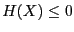• Type_Eq(i)=0 if equation i is a constraint equation of type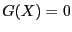• Type_Eq(i)=1 if equation i is a constraint equation of type• Type_Eq(i)=-2 if equation i is the optimum function to be minimized
• Type_Eq(i)=2 if equation i is the optimum function to be maximized
• Type_Eq(i)=10 if equation i is the optimum function for which is sought the minimum and maximum
• IntervalFunction: a function which return the interval vector evaluation of the equations, see the note 2.3.4.3. This function must be written in a similar manner than for the general solving procedures.
• Gradient: a function which return the interval evaluation of the gradient of the equations, see the note 2.4.2.2. This function must be written in a similar manner than for the general solving procedures with the additional constraint that the function to be minimized of maximized must be the last one.
• TheDomain: box in which we are looking for the extremum of the optimum function
• Iteration: the number of boxes that may be stored
• Order: a flag describing which order is used to store the new boxes, see the note 8.3.4
• epsilon: the maximal width of the solution intervals but not used. Should be set to 0.
• epsilonf: the maximal error for the equality constraints. If the problem has constraint of typethen a solution will verify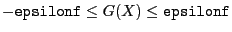• epsilone: the maximal error on the extremum value. If the extremum of the function is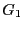and the procedure returns the value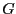, then a minimum will verify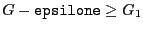and a maximum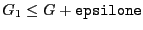.
• Func_Has_Interval: 1 if the optimum function has interval coefficients, 0 otherwise
• Optimum: an interval which contain the extremum value of the optimum function
• Solution: an interval matrix of size at least (2,m) which will contained the values of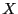for which the extremum are obtained
• Simp_Proc: an optional parameter which is a simplification procedure that may be provided by the user. It takes as input a box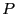and may:
• either returns ina box with lower width than the initialand a return code 0 or 1
• or indicates that there is no solution to the optimization problem in the current box, in which case the procedure returns -1
Thus to minimize a function you have to set its Type_Eq to -2 and to maximize it to set its Type_Eq to 2.

Remember that you may use the 3B method to improve the efficiency of this algorithm (see section 2.3.2).

Note also that a convenient way to write the IntervalFunction and Gradient procedures is to use the possibilities offered by ALIAS-Maple (see the ALIAS-Maple manual).Next: Return code Up: Implementation Previous: Dealing with inequalities on   Contents
Jean-Pierre Merlet 2012-12-20